Solving Pair of Linear Inequalities

Chapter 5 Class 11 Linear Inequalities
Serial order wise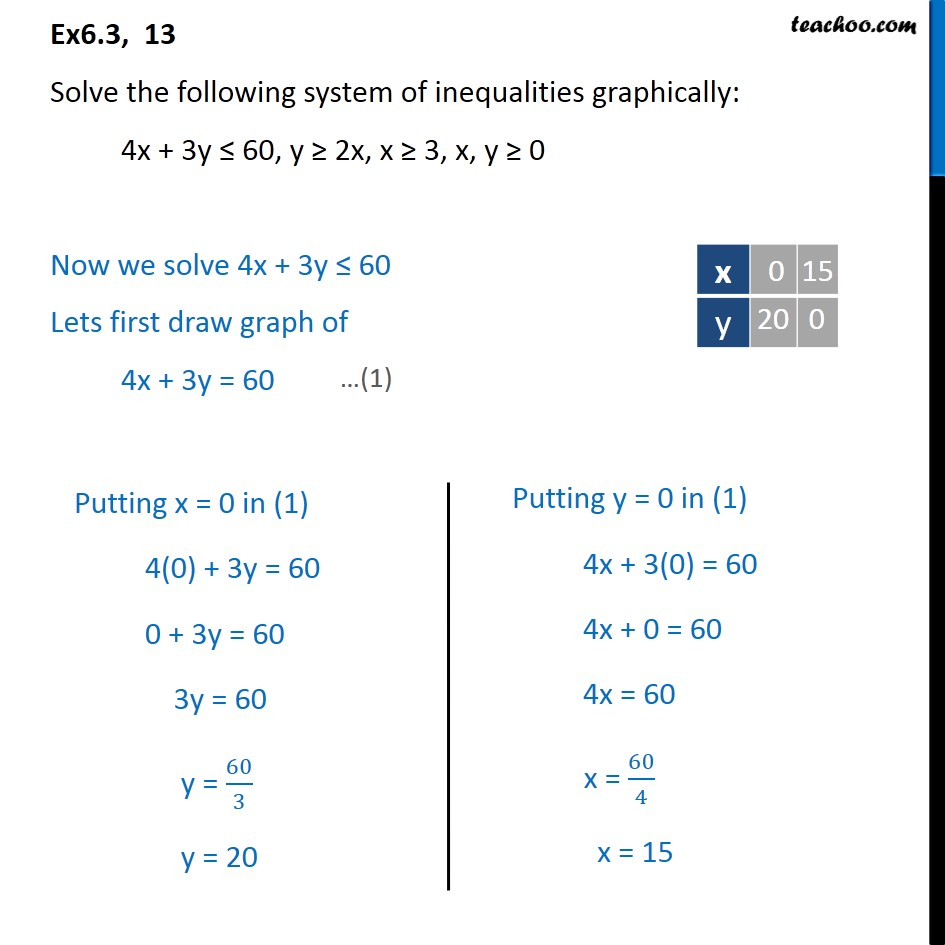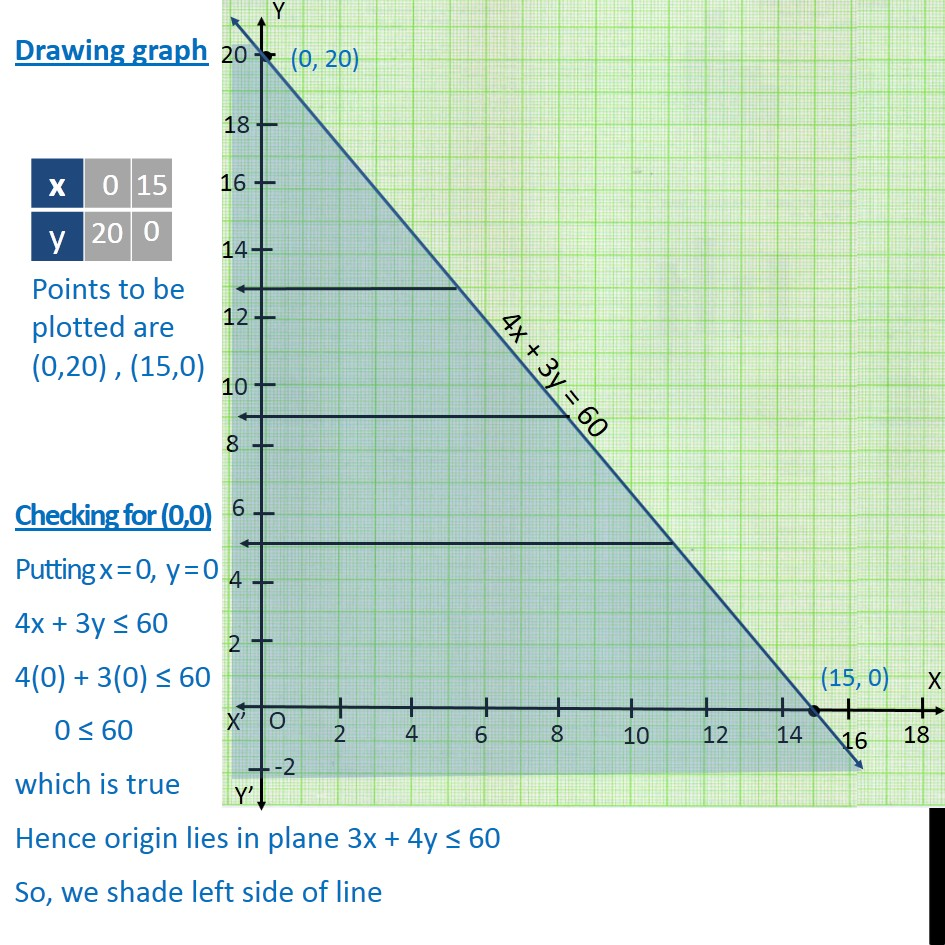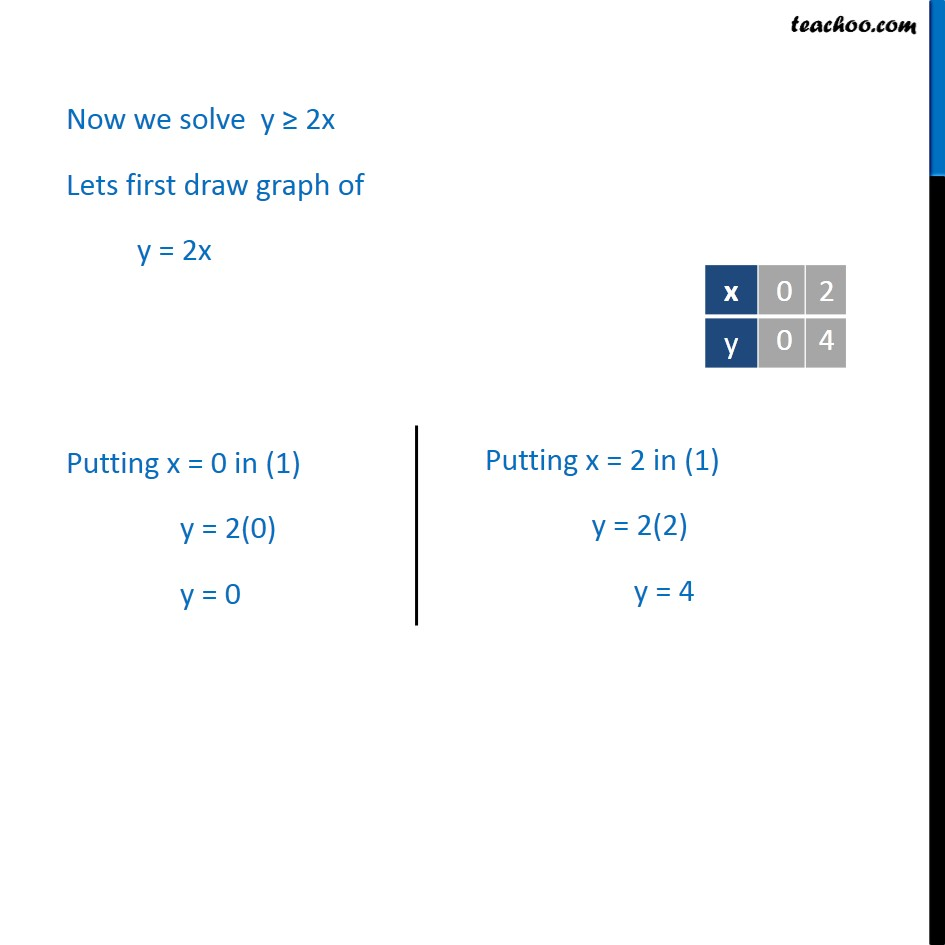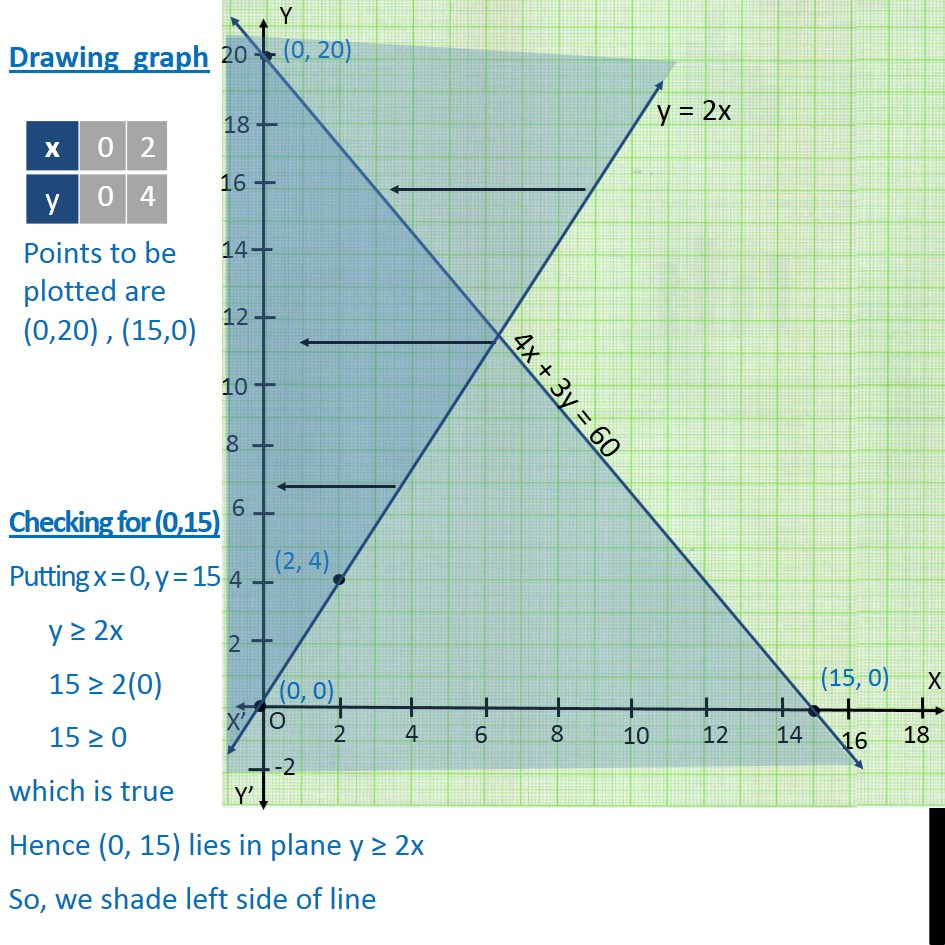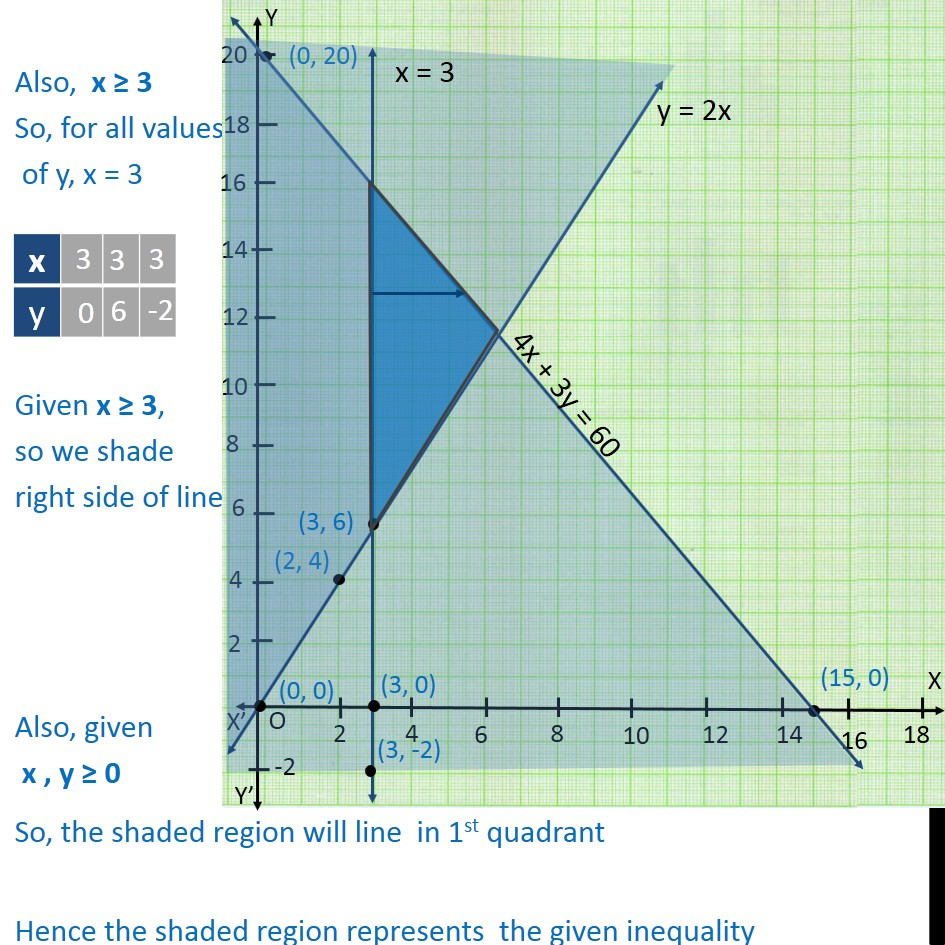Learn in your speed, with individual attention - Teachoo Maths 1-on-1 Class

### Transcript

Ex6.3, 13 Solve the following system of inequalities graphically: 4x + 3y ≤ 60, y ≥ 2x, x ≥ 3, x, y ≥ 0 Now we solve 4x + 3y ≤ 60 Lets first draw graph of 4x + 3y = 60 Drawing graph Checking for (0,0) Putting x = 0, y = 0 4x + 3y ≤ 60 4(0) + 3(0) ≤ 60 0 ≤ 60 which is true Hence origin lies in plane 3x + 4y ≤ 60 So, we shade left side of line Now we solve y ≥ 2x Lets first draw graph of y = 2x Drawing graph Checking for (0,15) Putting x = 0, y = 15 y ≥ 2x 15 ≥ 2(0) 15 ≥ 0 which is true Hence (0, 15) lies in plane y ≥ 2x So, we shade left side of line Also, x ≥ 3 So, for all values of y, x = 3 Given x ≥ 3, so we shade right side of line Also, given x , y ≥ 0 So, the shaded region will line in 1st quadrant Hence the shaded region represents the given inequality